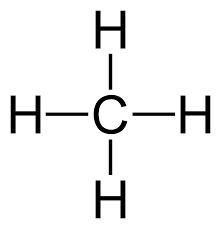# What is the oxidation state of Cr in K_2Cr_2O_7?

What is the oxidation state of Cr in K_2Cr_2O_7?

ReportAnswer 1

We have $C r \left(V I +\right)$, the metal in its maximum oxidation state, its Group oxidation state.

#### Explanation:

$\text{Oxidation state/number}$ is the charge left on the central atom, when all the bonding atoms are removed with the charge devolved to the more electronegative atom. The sum of the oxidation numbers of each species is the charge on the ion.

We have the $C {r}_{2} {O}_{7}^{2 -}$ anion, and, as is typical, each oxygen atom has a formal $- I I$ oxidation number: thus $7 \times \left(- I I\right) + 2 \times C {r}_{\text{oxidation number}} = - 2$. Clearly, $C {r}_{\text{oxidation number}} = V I +$.

Because, chromium is in such a high oxidation state, it typically accepts electron density from other reagents, thereby oxidizing them, and reducing itself. Dichromate is thus a good oxidizing agent. Typically we see the colour change from red/orange, $C r \left(V I +\right)$, to green $C r \left(I I I +\right)$:

$C {r}_{2} {O}_{7}^{2 -} + 14 {H}^{+} + 6 {e}^{-} \rightarrow 2 C {r}^{3 +} + 7 {H}_{2} O$

Are charge and mass balanced here? They should be.

##### Add Answer of: What is the oxidation state of Cr in K_2Cr_2O_7?
Similar Homework Help Questions

• ### What is the C oxidation state, O oxidation state, and the H oxidation state?• ### What is the oxidation state

A vanadium electrode is oxidized electrically. If the mass ofthe electrode decreases by 114 mg during the passage of 650coulombs, what is the oxidation state ofthe vanadiumproduct?A. +1B. +2C. +3D. +4Please EXPLAIN youranswer.

• ### What is the oxidation state of the metal?

What is the oxidation state of the metal in each of the following compounds:a)(NH4)3[RhCl6]b)[Cr(NH3)4(SCN)2]Brc)[Cu(en)2]SO4d)Na2[Mn(EDTA)]

• ### What is the oxidation state of nitrogen?

Need Online Homework Help?